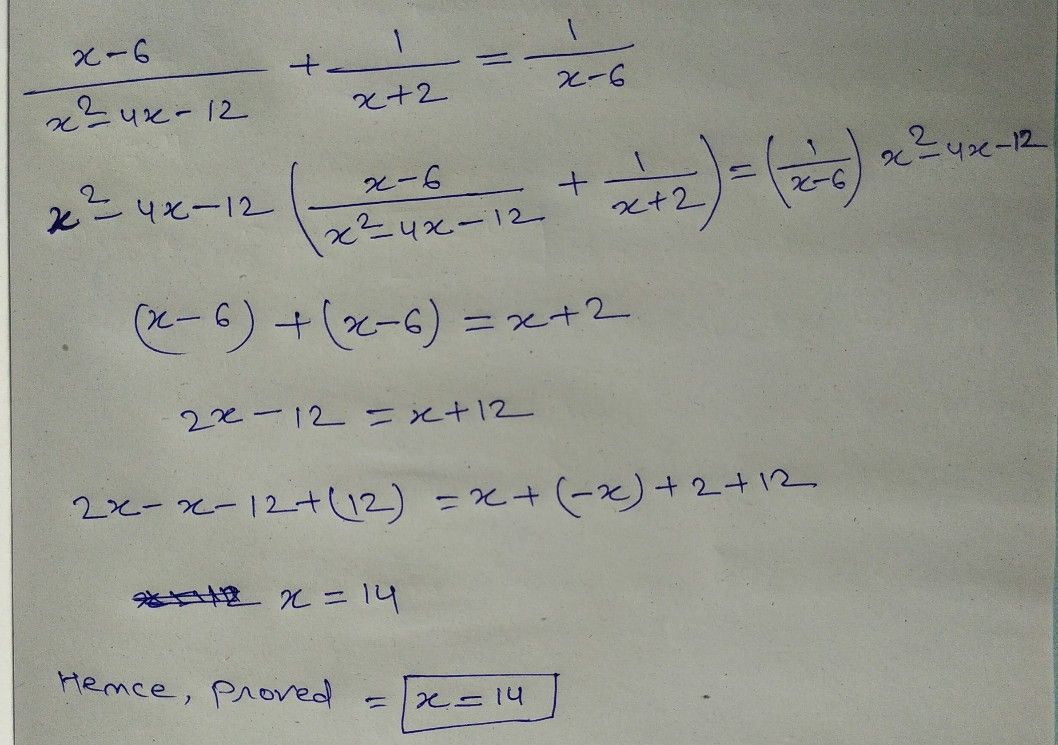Symbol
Problem$2$ $\dfrac {x-6} {x^{2}-4x-12}+\dfrac {2} {x+2}=\dfrac {1} {x-6}$ Rational Equation 1. Find the Least Common Denominator (LCD). $2$ Multiply both sides of the equation by its the LCD. $3$ Apply the Distributive Property and then simplify $4$ Find all the possible values of $x$ $x=10$ $50$ Check each value by substituting into original equation and reject any extraneous $oot/s$
10th-13th grade
Algebra
Search count: 111
SolutionQanda teacher - beb